### 【原】MATALB结合的多层分析应用服务。

MS-SQL Server > SQL Server BI [问题点数：200分，结帖人MSTOP]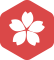2004年3月 VB大版内专家分月排行榜第一2004年3月 VB大版内专家分月排行榜第一2004年3月 VB大版内专家分月排行榜第一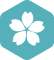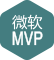2004年3月 VB大版内专家分月排行榜第一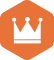2012年 荣获名人称号2010年 总版技术专家分年内排行榜第九
2009年 总版技术专家分年内排行榜第八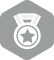2011年9月 总版技术专家分月排行榜第二
2009年11月 总版技术专家分月排行榜第二2004年3月 VB大版内专家分月排行榜第一
CSDN计算机类推荐帖和自我感觉很好的帖

Matlab 图像处理-哈夫曼编码（huffman）

MATALB仿真架构：源比特 +卷积码 +BPSK +AWGN +Viterbi +BER  说明：卷积编码，不同的R码率，不同的约束长度 2. Turbo增益性能、误码率  信道环境：AWGN  信噪比SNR ：-1:0.1:2  MATALB

y = gaussmf(x,[sig c]) 其中，c是位置参数，sig是尺度参数，控制图形的胖瘦。x = 0:0.1:10; y = gaussmf(x,[2 5]); plot(x,y) xlabel('gaussmf, P=[2 5]')参考

Matlab hist（）函数

histogram是一个在图像上应用广泛的一个统计工具，bag-of-word，spatial pymarid matching等等都是基于histogram的。那么如何去做到有效的统计，而不去自己写很长很慢的循环完成这项工作呢？matlab提供了一个非常...

【Tools】Matlab2015b关联m文件方法

MATLAB中字符串数组的文件输出

MATLAB是用二维数组来保存字符串数组的。将一个字符串数组加一列实数数组，输出到文件。 clear clc %字符串数组 str = [ '01-May-1998 01:00:00' '01-May-1998 01:10:00' '01-May-1998 01:20:00' ...'01-May-199

Matlab中Robotics toolbox的安装及使用

Matlab实现直方图均衡化

Matlab实现直方图均衡化 G=imread('aini555.jpg'); I=rgb2gray(G); J=histeq(I); %直方图均衡化，这一个函数就可以做到均衡化的效果 ...title('图') subplot(122),imshow(ui...

Matlab函数创建与调用

function [ out] = name( in ) …… …… return end 以上，out代表函数输出，in代表函数输入，end代表结束，name代表函数名。 ...调用的时候，如果在同一个文件夹，可以直接以下方式调用 ...如果不在同一个文件夹，可以...

Matlab矩阵行相加、列相加

Z=[1 2;3 4] b=sum(Z) %将列相加 c=sum(Z,2) %将行相加 运行结果：  Z =  1 2  3 4 b =  4 6 c =  3  7

2、如果没有链接Matalb会自动打开如下界面 3、如果你安装了Matlab，会自动检测出Matlab的版本。点击OK就可以。如果安装了Matlab，而没有检测出来，就需要手动添加Matlab。点击Find Matlab，到安装Matlab的目录去...

r = corr2(A,B)

MATLAB--相机标定教程

MATLAB repmat函数的使用

repmat函数repmat函数主要是用于快速的产生一个大的矩阵，它的用法一般有下面几种：B = repmat(A,M,N)矩阵B是矩阵A的复制品，其中B的维度为[size(A,1)*M, size(A,2)*N]。如果你写成repmat(A,[M,N])也是可以的；...

Matlab中.*和./详解

1.概念 .*和./用于矩阵相乘或者矩阵相除，分别表示矩阵中对应元素相乘和对应元素相除。 2.实例：

Matlab之画图实例

%在MATALB计算生成的图片上标出图名和最大值点坐标; %该函数公式为 y=exp(-2*t).*sin(3*t); 0 实例： a=2; b=3; t=0:0.01:10; y=exp(-a*t).*sin(b*t); [y_max,i_max]=max(y); t_text=['t=',num2str(t(i_max))];%...

MATLAB矩阵的基本运算及操作

matlab 显示系统当前时间

MATLAB描绘饼图——pie

MatLab 中计算开根号

1. 方法 例如 如果对4要开根号 可以输入函数如下 >> sqrt(4) 2方法 根号其实是1/2次方 >> 4^(1/2) 同样可以得到结果

Matlab中的ispc命令

【matlab 数据处理】excel读取和写出，匹配

matlab中的numel函数

MATLAB求和函数sum/cumsum

MATLAB求和函数sum/cumsum